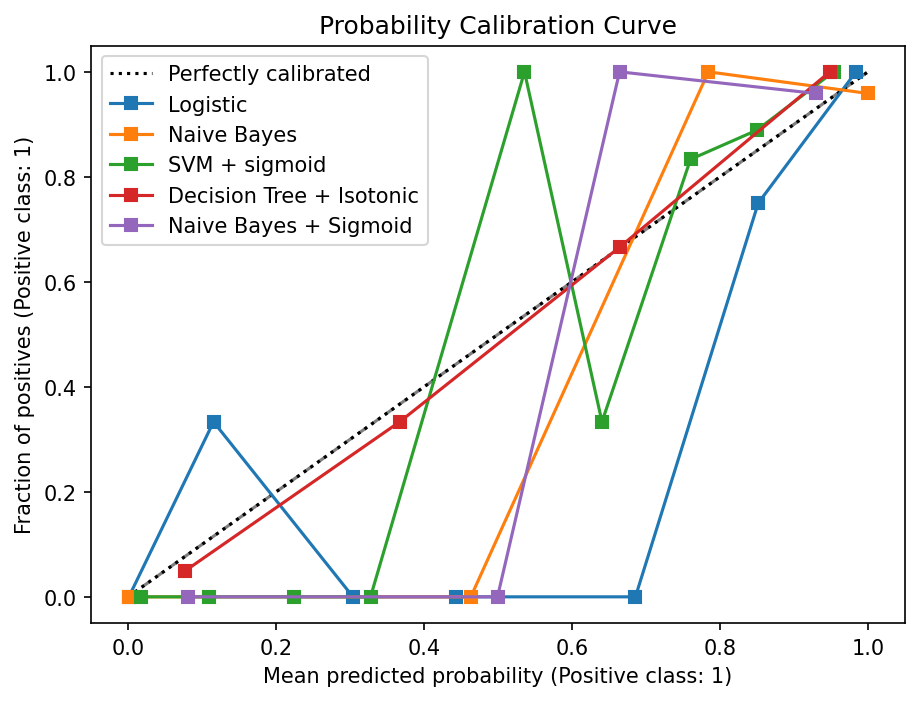# Probability Calibration of Classifiers in Scikit Learn

In this article, we will explore the concepts and techniques related to the probability calibration of classifiers in the context of machine learning. Classifiers in machine learning frequently provide probabilities indicating how confident they are in their predictions. However, the probabilities may not be well-calibrated. This means they do not accurately represent the true likelihood of the predicted class. To obtain well-calibrated probabilities, output probabilities are mapped through probability calibration. The Scikit-learn library includes techniques for improving classifier accuracy through probability calibration.

The process of determining how accurately the classification model’s estimated probabilities correspond to the actual probabilities of the events being predicted is known as probability calibration.

### There are two common approaches to probability calibration in Scikit-learn:

1. Platt Scaling (Logistic Regression) method: Fitting a logistic regression model to the classifier’s output probabilities is accomplished by this method. A calibrated probability mapping function from the original probabilities is established by using maximum likelihood estimation. With the assistance of logistic regression, Scikit-learn’s CalibratedClassifierCV class facilitates probability calibration.

2. Isotonic Regression: The true probabilities are believed to be monotonically (mathematical concept where a function or sequence either consistently increases or consistently decreases) related to the original ones in this method. The classifier’s output probabilities are fit with a non-parametric isotonic regression model. A function that increases monotonically is learned by this model to map the original probabilities to calibrated probabilities. Scikit learns Isotonic Regression class is useful for achieving isotonic calibration.

The command to install scikit-learn using pip is:

`pip install scikit-learn`

Example 1: In this code, we will binary classification problem to demonstrate probability calibration using Platt Scaling in scikit-learn.

## Python3

 `from` `sklearn.datasets ``import` `load_iris` `from` `sklearn.model_selection ``import` `train_test_split` `from` `sklearn.linear_model ``import` `LogisticRegression` `from` `sklearn.calibration ``import` `CalibratedClassifierCV` `import` `numpy as np`   `# Load the Iris dataset` `iris ``=` `load_iris()` `X ``=` `iris.data` `y ``=` `iris.target`   `# Split the dataset into training and testing sets` `X_train, X_test, y_train, y_test ``=` `train_test_split(X, y, test_size``=``0.2``, random_state``=``42``)`   `# Train a logistic regression classifier` `clf ``=` `LogisticRegression()` `clf.fit(X_train, y_train)`   `# Create a calibrated classifier using Platt Scaling` `calibrated_clf ``=` `CalibratedClassifierCV(clf, method``=``'sigmoid'``)` `calibrated_clf.fit(X_train, y_train)`   `# Make predictions on the testing set` `y_proba ``=` `calibrated_clf.predict_proba(X_test)`   `# Print the predicted class labels and probabilities` `print``(``"Predicted Probabilities:\n"``,[np.argmax(prob) ``for` `prob ``in` `y_proba])`

Output:

```Predicted Probabilities:
[2, 0, 2, 1, 2, 0, 1, 2, 2, 1, 2, 0, 0, 0, 0, 2, 2, 1, 1, 2, 0, 2, 0, 2, 2, 2, 2, 2, 0, 0]```

Explanation: Functions used in the above code are:

• train_test_split():  to split the dataset into training and testing sets
• LogisticRegression(): as the base classifier
• CalibratedClassifierCV(): for probability calibration

iris dataset is first loaded and the features and target variables are assigned to X and Y, respectively. Next, the dataset is split into training and testing sets using the train_test_split function. Here, 80% of the data is used for training (X_train, y_train), and 20% is used for testing (X_test, y_test).

Now, calibrated_clf is been created, and the calibrated classifier is then trained using the training data. Next, predictions are made on the testing set using the calibrated classifier. The predicted probabilities for each class are stored in y_proba.

Finally, the predicted class labels and probabilities are printed.

• predicted labels (y_pred) represent the class predictions for each sample in the testing set
• predicted probabilities (y_proba) show the probability estimates for each class

## Python3

 `from` `sklearn.datasets ``import` `load_breast_cancer` `from` `sklearn.model_selection ``import` `train_test_split` `from` `sklearn.svm ``import` `SVC` `from` `sklearn.tree ``import` `DecisionTreeClassifier` `from` `sklearn.linear_model ``import` `LogisticRegression` `from` `sklearn.naive_bayes ``import` `GaussianNB` `from` `sklearn.calibration ``import` `CalibratedClassifierCV` `from` `sklearn.calibration ``import` `CalibrationDisplay` `import` `matplotlib.pyplot as plt`   `# Load the Breast Cancer dataset` `cancer ``=` `load_breast_cancer()` `X ``=` `cancer.data` `y ``=` `cancer.target`   `# Split the dataset into training and testing sets` `X_train, X_test, y_train, y_test ``=` `train_test_split(X, y, test_size``=``0.2``, random_state``=``42``)`   `# Classifiers` `svc ``=` `SVC()` `tree ``=` `DecisionTreeClassifier()` `log ``=` `LogisticRegression(C``=``0.5``)` `gnb ``=` `GaussianNB()` `svc_sigmoid ``=` `CalibratedClassifierCV(svc, cv``=``3``, method``=``"sigmoid"``, ensemble``=``True``)` `tree_isotonic ``=` `CalibratedClassifierCV(tree, cv``=``3``, method``=``"isotonic"``, ensemble``=``True``)` `gnb_sigmoid ``=` `CalibratedClassifierCV(gnb, cv``=``3``, method``=``"sigmoid"``, ensemble``=``True``)`   `classifiers ``=` `{` `    ``"Logistic"``:log,` `    ``"Naive Bayes"` `: gnb,` `    ``"SVM + sigmoid"``: svc_sigmoid,` `    ``"Decision Tree + Isotonic"``: tree_isotonic,` `    ``"Naive Bayes + Sigmoid"` `: gnb_sigmoid` `}`   `# Plot Probability Calibration curve for each classifier` `fig, ax ``=` `plt.subplots(figsize``=``(``7``, ``5``), dpi``=``150``)` `ax.plot([``0``, ``1``], [``0``, ``1``], linestyle``=``'--'``, color``=``'gray'``)`   `for` `name, clf ``in` `classifiers.items():` `    ``clf.fit(X_train, y_train)` `    ``clf_disp ``=` `CalibrationDisplay.from_estimator(clf, X_test, y_test, n_bins``=``10``, name``=``name, ax``=``ax)`   `plt.title(``'Probability Calibration Curve'``)` `plt.legend(loc``=``"best"``)` `plt.show()`

Output:Probability Calibration Curve

Whether you're preparing for your first job interview or aiming to upskill in this ever-evolving tech landscape, GeeksforGeeks Courses are your key to success. We provide top-quality content at affordable prices, all geared towards accelerating your growth in a time-bound manner. Join the millions we've already empowered, and we're here to do the same for you. Don't miss out - check it out now!

Previous
Next